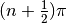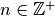# Binary Hamiltonian Monte Carlo (BHMC)¶

Implementation of the binary-state Hamiltonian Monte Carlo sampler of Pakman . The sampler simulates autocorrelated draws from a distribution that can be specified up to a constant of proportionality.

## Model-Based Constructor¶

BHMC(params::ElementOrVector{Symbol}, traveltime::Real)

Construct a Sampler object for BHMC sampling. Parameters are assumed to have binary numerical values (0 or 1).

Arguments

• params : stochastic node(s) to be updated with the sampler.
• traveltime : length of time over which particle paths are simulated. It is recommended that supplied values be of the form, where optimal choices ofare expected to grow with the parameter space dimensionality.

Value

Returns a Sampler{BHMCTune} type object.

Example

See the Pollution and other Examples.

## Stand-Alone Function¶

sample!(v::BHMCVariate)

Draw one sample from a target distribution using the BHMC sampler. Parameters are assumed to have binary numerical values (0 or 1).

Arguments

• v : current state of parameters to be simulated.

Value

Returns v updated with simulated values and associated tuning parameters.

Example

################################################################################
## Linear Regression
##   y ~ MvNormal(X * (beta0 .* gamma), 1)
##   gamma ~ DiscreteUniform(0, 1)
################################################################################

using Mamba

## Data
n, p = 25, 10
X = randn(n, p)
beta0 = randn(p)
gamma0 = rand(0:1, p)
y = X * (beta0 .* gamma0) + randn(n)

## Log-transformed Posterior(gamma) + Constant
logf = function(gamma::DenseVector)
logpdf(MvNormal(X * (beta0 .* gamma), 1.0), y)
end

## MCMC Simulation with Binary Hamiltonian Monte Carlo Sampling
t = 10000
sim = Chains(t, p, names = map(i -> "gamma[\$i]", 1:p))
gamma = BHMCVariate(zeros(p), (2 * p + 0.5) * pi, logf)
for i in 1:t
sample!(gamma)
sim[i, :, 1] = gamma
end
describe(sim)


## BHMCVariate Type¶

### Declaration¶

const BHMCVariate = SamplerVariate{BHMCTune}

### Fields¶

• value::Vector{Float64} : simulated values.
• tune::BHMCTune : tuning parameters for the sampling algorithm.

### Constructor¶

BHMCVariate(x::AbstractVector{T<:Real}, traveltime::Real, logf::Function)

Construct a BHMCVariate object that stores simulated values and tuning parameters for BHMC sampling.

Arguments

• x : initial values.
• traveltime : length of time over which particle paths are simulated. It is recommended that supplied values be of the form, where optimal choices ofare expected to grow with the parameter space dimensionality.
• logf : function that takes a single DenseVector argument of parameter values at which to compute the log-transformed density (up to a normalizing constant).

Value

Returns a BHMCVariate type object with fields set to the supplied x and tuning parameter values.

## BHMCTune Type¶

### Declaration¶

type BHMCTune <: SamplerTune

### Fields¶

• logf::Nullable{Function} : function supplied to the constructor to compute the log-transformed density, or null if not supplied.
• traveltime::Float64 : length of time over which particle paths are simulated.
• position::Vector{Float64} : initial particle positions.
• velocity::Vector{Float64} : initial particle velocites.
• wallhits::Int : number of times particles are reflected off the 0 threshold.
• wallcrosses::Int : number of times particles travel through the threshold.